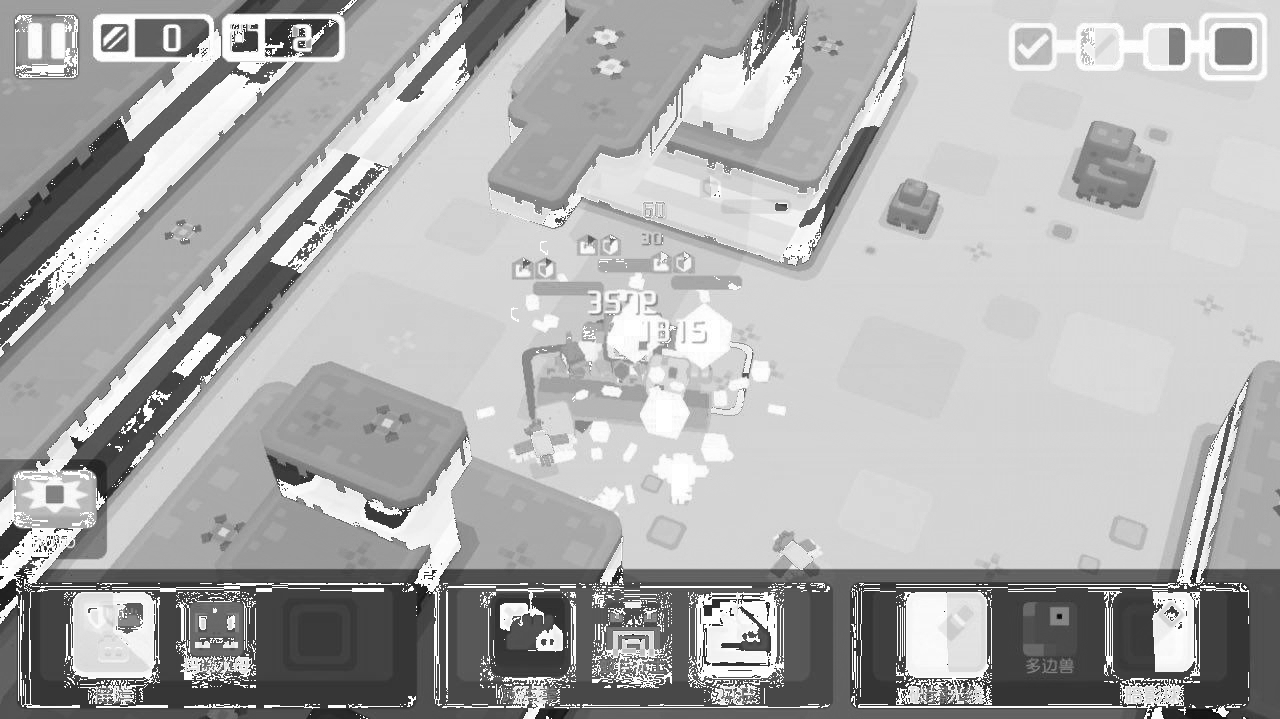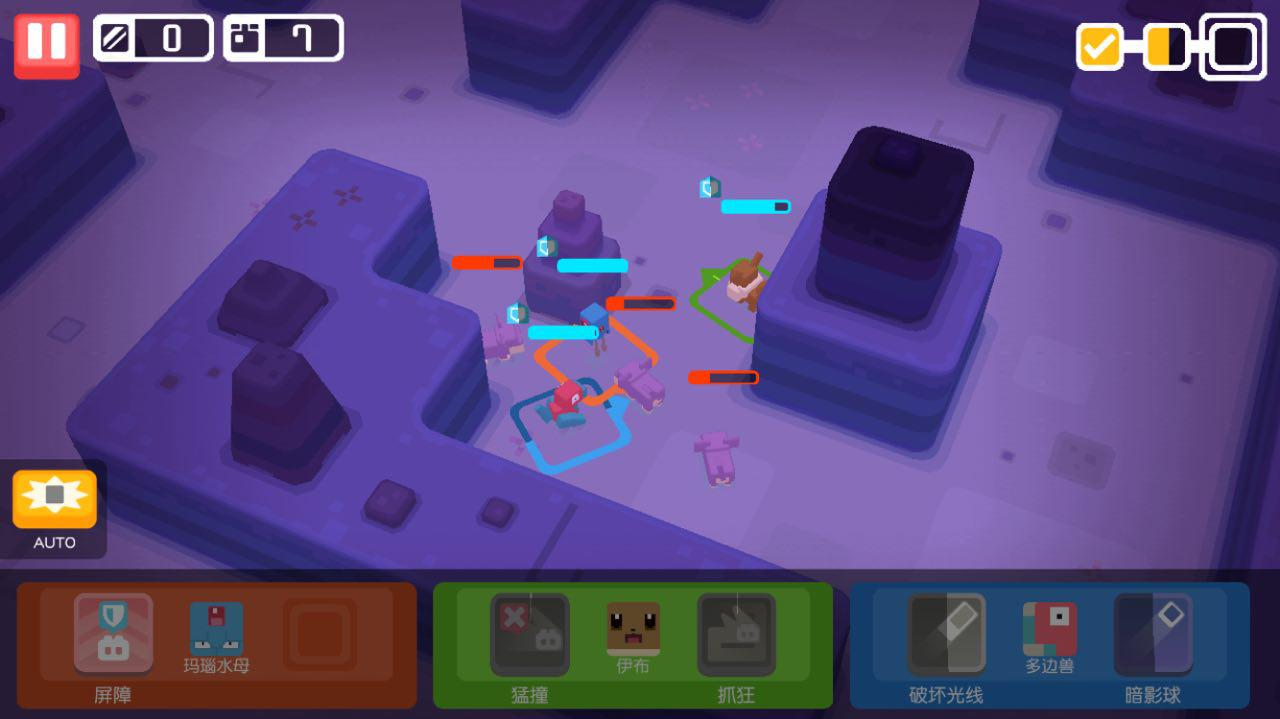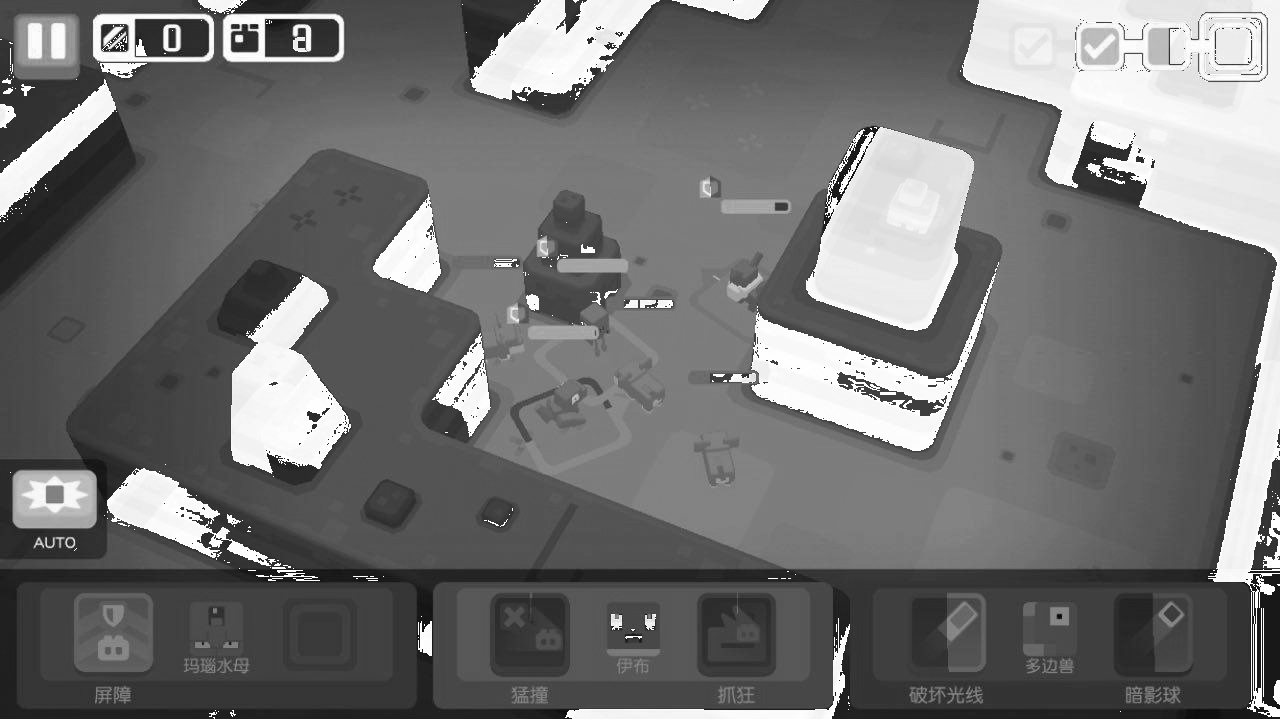# 幻影坦克1. 通过棋盘格交错显示
2. 通过对黑白背景下的图片进行计算后合成新图片

$$Gray=Gray_{Front} \times Alpha \div 255 + Gray_{Back} \times (255 – Alpha)\div 255$$

$$\begin{cases} Gray_{Front} &= Gray_{White} \\ Gray_{Back} &=255 \end{cases}$$

$$\begin{cases} Gray_{Front} &= Gray_{Black} \\ Gray_{Back} &=0 \end{cases}$$

$$\begin{cases} Alpha &= 255 + Gray_{Black} – Gray_{White} \\ Gray &=255 \times Gray_{Black}/Alpha \end{cases}$$

from PIL import Image
import sys
import numpy as np

def main(argv):
if len(argv) != 4:
print("Error")
pass

image_white = np.array(Image.open(argv).convert("L"))
image_black = np.array(Image.open(argv).convert("L"))
image_black = image_black * 0.3

a = 255.0 + image_black - image_white
g = 255.0 / a * image_black
g[g == np.inf] = 255.0
g.clip(0, 255)
a.clip(0, 255)

a = np.round(a, 0).astype("ubyte")
g = np.round(g, 0).astype("ubyte")

print("amax:%d gmax%d amin:%d gmin:%d" % (a.max(), g.max(), a.min(), g.min()))

result = np.dstack([g, g, g, a])

print(result.shape)

im = Image.fromarray(result, "RGBA")

im.save(argv, "PNG")
im.show()

if __name__ == '__main__':
main(sys.argv)## One thought on “幻影坦克”

1.Kaixin Xu说道：

带我飞